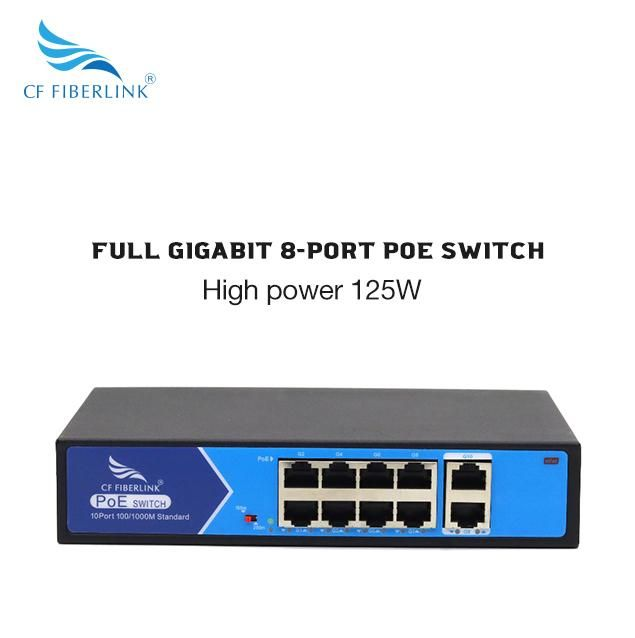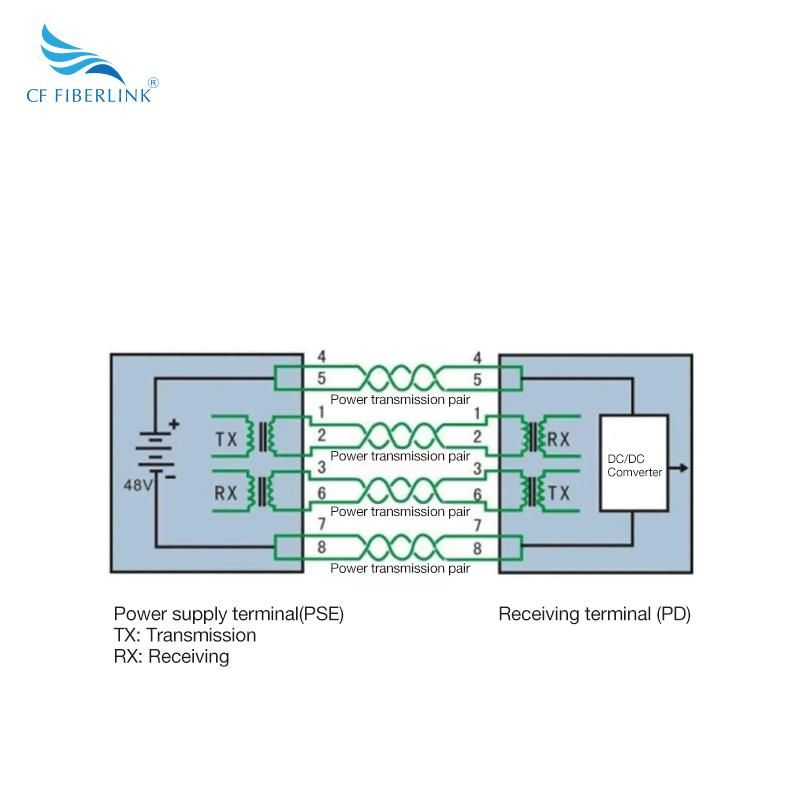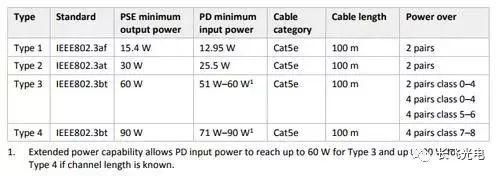•# CF FIBERLINK – Teach you a detailed understanding of POE power supply issues!

Many friends have repeatedly asked whether PoE's power supply is stable? What cable is good for PoE power supply? Why does the camera still not display when powered by a PoE switch? And so on, these are actually related to the power loss of POE power supply, which is easily overlooked in projects.1、 What is POE power supply
PoE refers to the technology of providing DC power supply for some IP-based terminals (such as IP phones, wireless local area network access point APs, network cameras, etc.) without any modifications to the existing Ethernet Cat. 5 cabling infrastructure.
PoE technology can ensure the security of existing structured cabling while ensuring the normal operation of existing networks, minimizing costs.
A complete PoE system includes two parts: the power supply end device and the receiving end device.Power Supply Equipment (PSE): Ethernet switches, routers, hubs, or other network switching devices that support POE functionality.
Power receiving device (PD): In the monitoring system, it is mainly a network camera (IPC).
2、 POE power supply standard
The latest international standard IEEE802.3bt has two requirements:
The first type: One of them requires the PSE to achieve an output power of 60W, with a power reaching the receiving device of 51W (as shown in the table above, this is the lowest data), and a power loss of 9W.
The second method requires the PSE to achieve an output power of 90W, with a power of 71W reaching the receiving device and a power loss of 19W.
From the above standards, it can be seen that as the power supply increases, the loss of power is not proportional to the power supply, but rather increases. So how can the loss of PSE be calculated in practical applications?
3、 POE power supply loss
So let's first take a look at how middle school physics calculates the loss of wire power.
Joule's law is a law that quantitatively explains the conversion of electrical energy into thermal energy by conducting current.
The content is: The heat generated by the current passing through the conductor is proportional to the quadratic power of the current, the resistance of the conductor, and the time of electrification. That is, the personnel consumption generated during the calculation process.
Joule's law mathematical expression: Q=I ² Rt (applicable to all circuits), where Q is the power loss P, I is the current, R is the resistance, and t is the time.
In practical use, as PSE and PD work simultaneously, the loss is independent of time. The conclusion is that in a POE system, the loss power of the network cable is directly proportional to the quadratic power of the current and directly proportional to the size of the resistance. Simply put, in order to reduce the power consumption of the network cable, we should try to reduce the current of the wire and the resistance of the network cable as much as possible. The significance of reducing the current is particularly important.
So let's take a look at the specific parameters of international standards:
In the IEEE802.3af standard, the resistance of the network cable is 20 Ω, the required PSE output voltage is 44V, the current is 0.35A, and the loss power P=0.35 * 0.35 * 20=2.45W.
Similarly, in the IEEE802.3at standard, the resistance of the network cable is 12.5 Ω, the required voltage is 50V, the current is 0.6A, and the loss power P=0.6 * 0.6 * 12.5=4.5W.
There is no problem using this calculation method for both standards. But when it comes to the IEEE802.3bt standard, it cannot be calculated like this. If the voltage is 50V and the power to reach 60W needs to be 1.2A current, then the loss power is P=1.2 * 1.2 * 12.5=18W. Subtracting the loss, the power to reach the PD device is only 42W.
4、 Reasons for power loss in POE
So what exactly is the reason?
The actual requirement of 51W is reduced by 9W of electrical energy. So what exactly caused the calculation error.Power Supply Equipment (PSE): Ethernet switches, routers, hubs, or other network switching devices that support POE functionality.
Power receiving device (PD): In the monitoring system, it is mainly a network camera (IPC).
2、 POE power supply standard
The latest international standard IEEE802.3bt has two requirements:
The first type: One of them requires the PSE to achieve an output power of 60W, with a power reaching the receiving device of 51W (as shown in the table above, this is the lowest data), and a power loss of 9W.
The second method requires the PSE to achieve an output power of 90W, with a power of 71W reaching the receiving device and a power loss of 19W.
From the above standards, it can be seen that as the power supply increases, the loss of power is not proportional to the power supply, but rather increases. So how can the loss of PSE be calculated in practical applications?
3、 POE power supply loss
So let's first take a look at how middle school physics calculates the loss of wire power.
Joule's law is a law that quantitatively explains the conversion of electrical energy into thermal energy by conducting current.
The content is: The heat generated by the current passing through the conductor is proportional to the quadratic power of the current, the resistance of the conductor, and the time of electrification. That is, the personnel consumption generated during the calculation process.
Joule's law mathematical expression: Q=I ² Rt (applicable to all circuits), where Q is the power loss P, I is the current, R is the resistance, and t is the time.
In practical use, as PSE and PD work simultaneously, the loss is independent of time. The conclusion is that in a POE system, the loss power of the network cable is directly proportional to the quadratic power of the current and directly proportional to the size of the resistance. Simply put, in order to reduce the power consumption of the network cable, we should try to reduce the current of the wire and the resistance of the network cable as much as possible. The significance of reducing the current is particularly important.
So let's take a look at the specific parameters of international standards:
In the IEEE802.3af standard, the resistance of the network cable is 20 Ω, the required PSE output voltage is 44V, the current is 0.35A, and the loss power P=0.35 * 0.35 * 20=2.45W.
Similarly, in the IEEE802.3at standard, the resistance of the network cable is 12.5 Ω, the required voltage is 50V, the current is 0.6A, and the loss power P=0.6 * 0.6 * 12.5=4.5W.
There is no problem using this calculation method for both standards. But when it comes to the IEEE802.3bt standard, it cannot be calculated like this. If the voltage is 50V and the power to reach 60W needs to be 1.2A current, then the loss power is P=1.2 * 1.2 * 12.5=18W. Subtracting the loss, the power to reach the PD device is only 42W.
4、 Reasons for power loss in POE
So what exactly is the reason?
The actual requirement of 51W is reduced by 9W of electrical energy. So what exactly caused the calculation error.

It can be seen that the better the cable, the smallest the resistance, according to the formula Q=I ² Rt, which means that the power loss during the power supply process is the least, so this is why it is necessary to use cables well. It is recommended to use Category 6 cables as a safer option.
As we mentioned above, the loss power formula, Q=I ² Rt, in order to minimize the loss between the PSE power supply terminal and the PD receiving equipment, the minimum current and resistance are required to achieve the best performance throughout the entire power supply process.